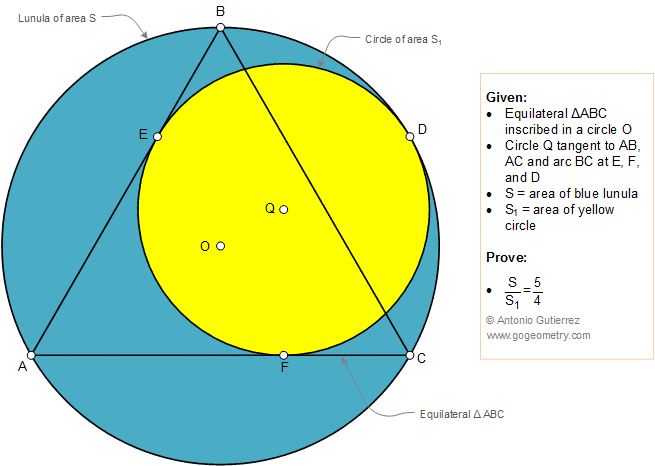# Geometry Problem 1362: Equilateral Triangle Inscribed in a Circle, Lunula, Ratio of Areas, Tangent, Sketch, iPad Apps.

### Proposition

The figure below shows an equilateral triangle ABC inscribed in a circle O. Circle Q is tangent to AB, AC, and arc BC at E, F, and D, respectively. S is the area of the blue lunula and S1 is the area of the yellow circle. Prove that S / S1 = 5 / 4.### Sketch and Typography of problem 1362 using iPad Apps